# 4.1 Linear functions  (Page 16/27)

 Page 16 / 27

Write an equation for a line perpendicular to $\text{\hspace{0.17em}}h\left(t\right)=-2t+4\text{\hspace{0.17em}}$ and passing through the point $\text{\hspace{0.17em}}\left(-4,–1\right).$

Write an equation for a line perpendicular to $\text{\hspace{0.17em}}p\left(t\right)=3t+4\text{\hspace{0.17em}}$ and passing through the point $\text{\hspace{0.17em}}\left(3,1\right).$

$y=-\frac{1}{3}t+2$

## Graphical

For the following exercises, find the slope of the line graphed.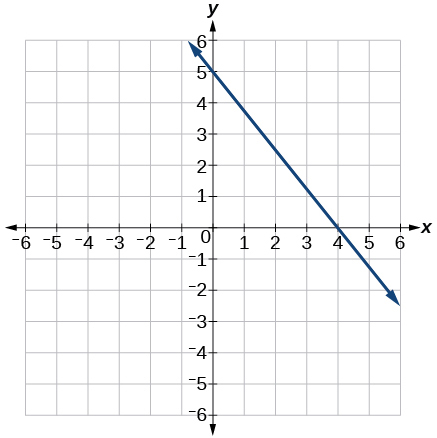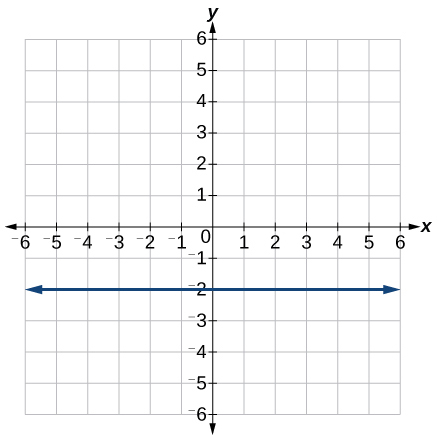0

For the following exercises, write an equation for the line graphed.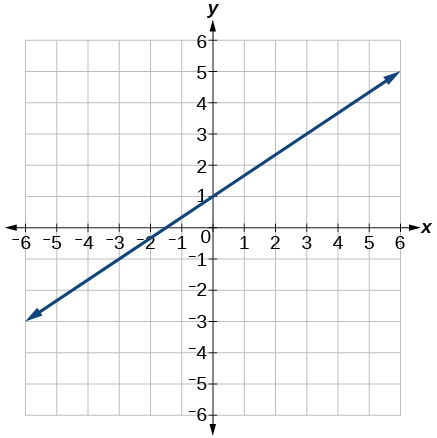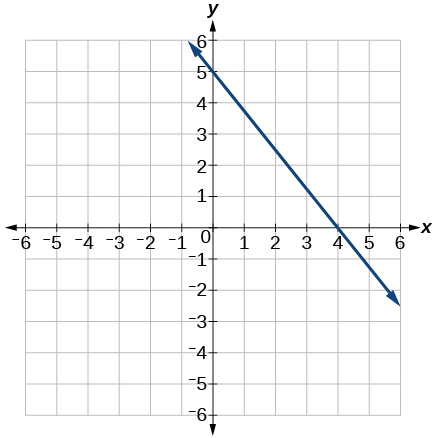$y=-\frac{5}{4}x+5$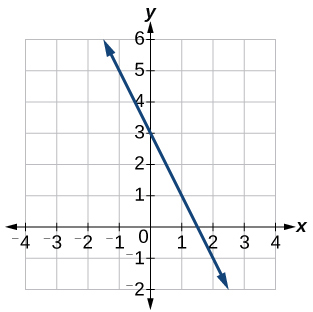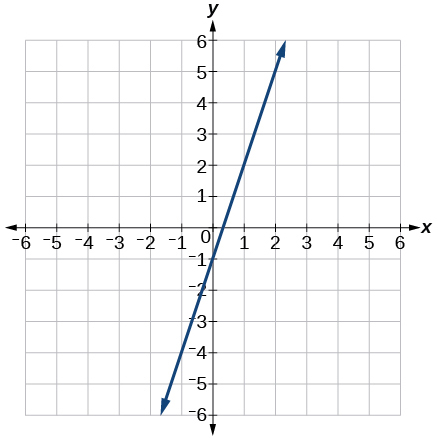$y=3x-1$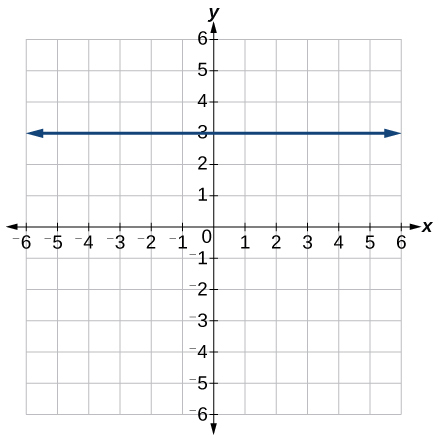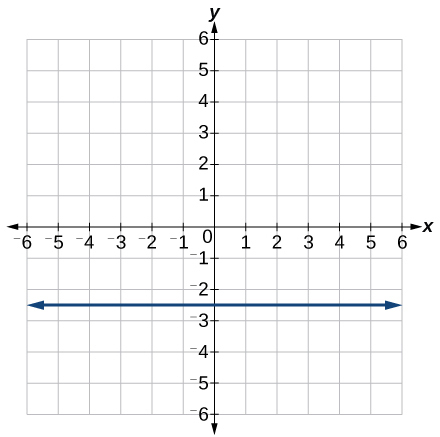$y=-2.5$

For the following exercises, match the given linear equation with its graph in [link] .

$f\left(x\right)=-x-1$

$f\left(x\right)=-2x-1$

F

$f\left(x\right)=-\frac{1}{2}x-1$

$f\left(x\right)=2$

C

$f\left(x\right)=2+x$

$f\left(x\right)=3x+2$

A

For the following exercises, sketch a line with the given features.

An x -intercept of $\text{\hspace{0.17em}}\left(–4,\text{0}\right)\text{\hspace{0.17em}}$ and y -intercept of $\text{\hspace{0.17em}}\left(0,\text{–2}\right)$

An x -intercept $\text{\hspace{0.17em}}\left(–2,\text{0}\right)\text{\hspace{0.17em}}$ and y -intercept of $\text{\hspace{0.17em}}\left(0,\text{4}\right)$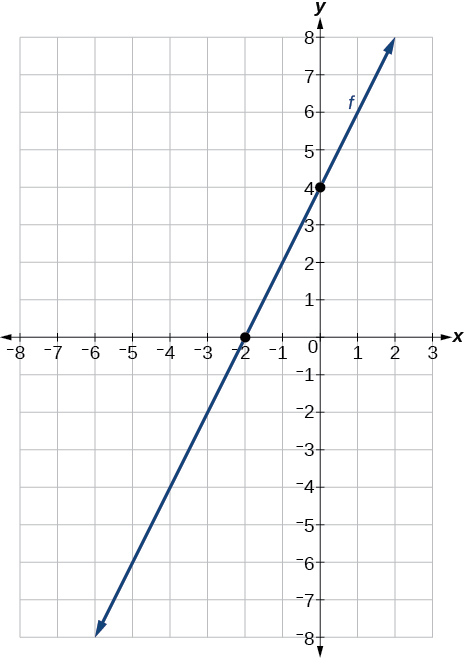A y -intercept of $\text{\hspace{0.17em}}\left(0,\text{7}\right)\text{\hspace{0.17em}}$ and slope $\text{\hspace{0.17em}}-\frac{3}{2}$

A y -intercept of $\text{\hspace{0.17em}}\left(0,\text{3}\right)\text{\hspace{0.17em}}$ and slope $\text{\hspace{0.17em}}\frac{2}{5}$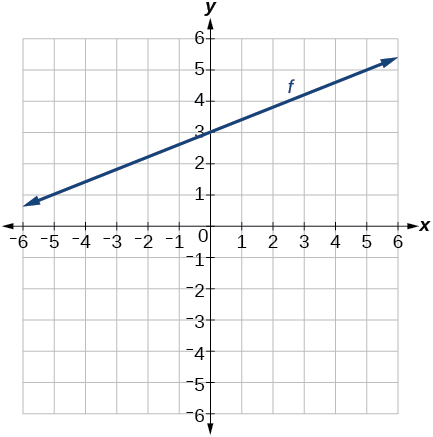Passing through the points $\text{\hspace{0.17em}}\left(–6,\text{–2}\right)\text{\hspace{0.17em}}$ and $\text{\hspace{0.17em}}\left(6,\text{–6}\right)$

Passing through the points $\text{\hspace{0.17em}}\left(–3,\text{–4}\right)\text{\hspace{0.17em}}$ and $\text{\hspace{0.17em}}\left(3,\text{0}\right)$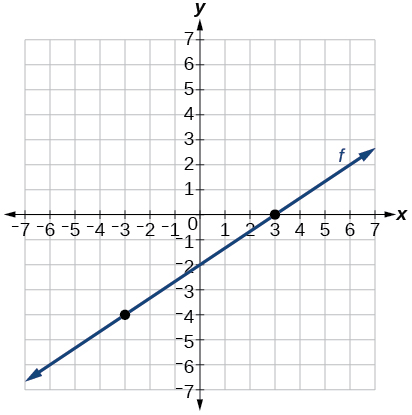For the following exercises, sketch the graph of each equation.

$f\left(x\right)=-2x-1$

$f\left(x\right)=-3x+2$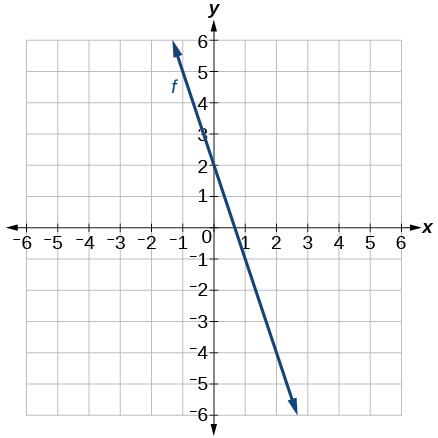$f\left(x\right)=\frac{1}{3}x+2$

$f\left(x\right)=\frac{2}{3}x-3$$f\left(t\right)=3+2t$

$p\left(t\right)=-2+3t$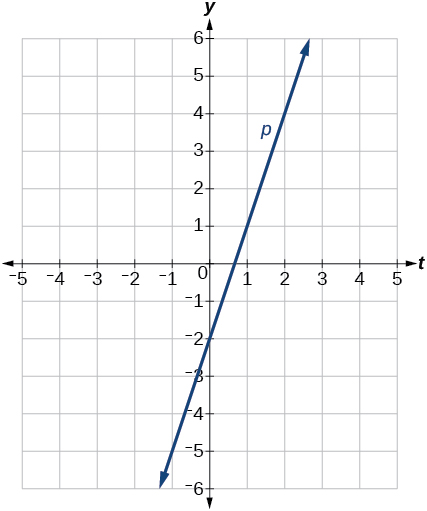$x=3$

$x=-2$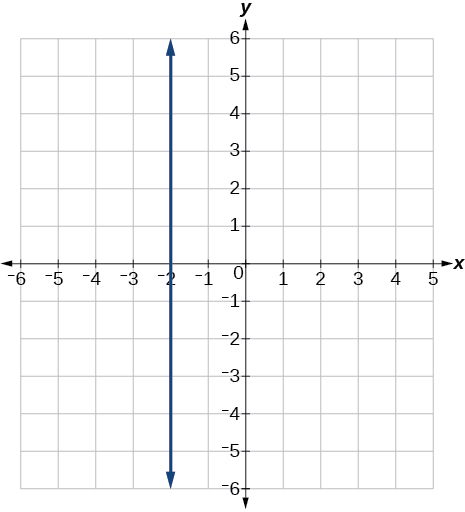$r\left(x\right)=4$

For the following exercises, write the equation of the line shown in the graph.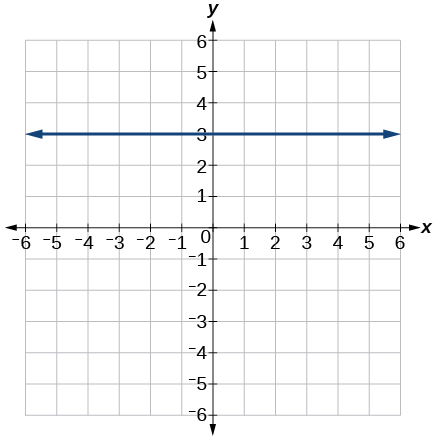$y=\text{3}$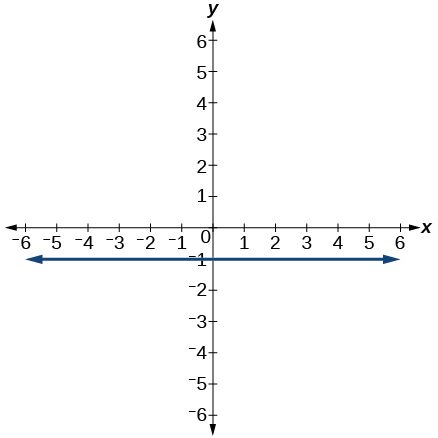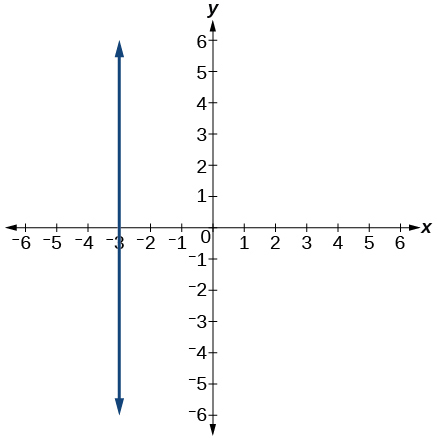$x=-3$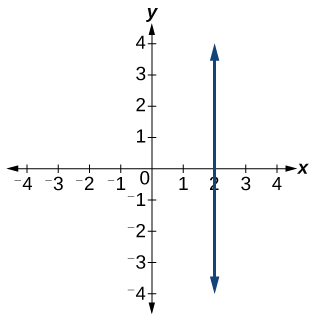## Numeric

For the following exercises, which of the tables could represent a linear function? For each that could be linear, find a linear equation that models the data.

 $x$ 0 5 10 15 $g\left(x\right)$ 5 –10 –25 –40

Linear, $\text{\hspace{0.17em}}g\left(x\right)=-3x+5$

 $x$ 0 5 10 15 $h\left(x\right)$ 5 30 105 230
 $x$ 0 5 10 15 $f\left(x\right)$ –5 20 45 70

Linear, $\text{\hspace{0.17em}}f\left(x\right)=5x-5$

 $x$ 5 10 20 25 $k\left(x\right)$ 13 28 58 73
 $x$ 0 2 4 6 $g\left(x\right)$ 6 –19 –44 –69

Linear, $\text{\hspace{0.17em}}g\left(x\right)=-\frac{25}{2}x+6$

 $x$ 2 4 8 10 $h\left(x\right)$ 13 23 43 53
 $x$ 2 4 6 8 $f\left(x\right)$ –4 16 36 56

Linear, $\text{\hspace{0.17em}}f\left(x\right)=10x-24$

 $x$ 0 2 6 8 $k\left(x\right)$ 6 31 106 231

## Technology

For the following exercises, use a calculator or graphing technology to complete the task.

If $\text{\hspace{0.17em}}f\text{\hspace{0.17em}}$ is a linear function, find an equation for the function.

$f\left(x\right)=-58x+17.3$

Graph the function $\text{\hspace{0.17em}}f\text{\hspace{0.17em}}$ on a domain of $\text{\hspace{0.17em}}\left[–10,10\right]:f\left(x\right)=0.02x-0.01.\text{\hspace{0.17em}}$ Enter the function in a graphing utility. For the viewing window, set the minimum value of $\text{\hspace{0.17em}}x\text{\hspace{0.17em}}$ to be $\text{\hspace{0.17em}}-10\text{\hspace{0.17em}}$ and the maximum value of $\text{\hspace{0.17em}}x\text{\hspace{0.17em}}$ to be $\text{\hspace{0.17em}}10.$

Graph the function $\text{\hspace{0.17em}}f\text{\hspace{0.17em}}$ on a domain of $\text{\hspace{0.17em}}\left[–10,10\right]:fx\right)=2,500x+4,000$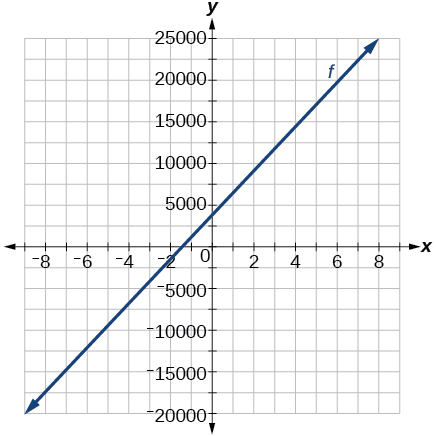[link] shows the input, $\text{\hspace{0.17em}}w,$ and output, $\text{\hspace{0.17em}}k,$ for a linear function $\text{\hspace{0.17em}}k.\text{\hspace{0.17em}}$ a. Fill in the missing values of the table. b. Write the linear function $\text{\hspace{0.17em}}k,$ round to 3 decimal places.

 w –10 5.5 67.5 b k 30 –26 a –44

[link] shows the input, $\text{\hspace{0.17em}}p,$ and output, $\text{\hspace{0.17em}}q,$ for a linear function $\text{\hspace{0.17em}}q.\text{\hspace{0.17em}}$ a. Fill in the missing values of the table. b. Write the linear function $\text{\hspace{0.17em}}k.$

 p 0.5 0.8 12 b q 400 700 a 1,000,000

Graph the linear function $\text{\hspace{0.17em}}f\text{\hspace{0.17em}}$ on a domain of $\text{\hspace{0.17em}}\left[-10,10\right]\text{\hspace{0.17em}}$ for the function whose slope is $\text{\hspace{0.17em}}\frac{1}{8}\text{\hspace{0.17em}}$ and y -intercept is $\text{\hspace{0.17em}}\frac{31}{16}.\text{\hspace{0.17em}}$ Label the points for the input values of $\text{\hspace{0.17em}}-10\text{\hspace{0.17em}}$ and $\text{\hspace{0.17em}}10.$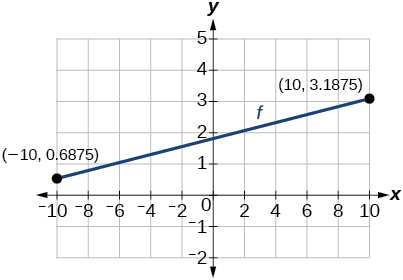Graph the linear function $\text{\hspace{0.17em}}f\text{\hspace{0.17em}}$ on a domain of $\text{\hspace{0.17em}}\left[-0.1,0.1\right]\text{\hspace{0.17em}}$ for the function whose slope is 75 and y -intercept is $\text{\hspace{0.17em}}-22.5.\text{\hspace{0.17em}}$ Label the points for the input values of $\text{\hspace{0.17em}}-0.1\text{\hspace{0.17em}}$ and $\text{\hspace{0.17em}}0.1.$

Graph the linear function $\text{\hspace{0.17em}}f\text{\hspace{0.17em}}$ where $\text{\hspace{0.17em}}f\left(x\right)=ax+b\text{\hspace{0.17em}}$ on the same set of axes on a domain of $\text{\hspace{0.17em}}\left[-4,4\right]\text{\hspace{0.17em}}$ for the following values of $\text{\hspace{0.17em}}a\text{\hspace{0.17em}}$ and $\text{\hspace{0.17em}}b.$

1. $a=2;b=3$
2. $a=2;b=4$
3. $a=2;b=–4$
4. $a=2;b=–5$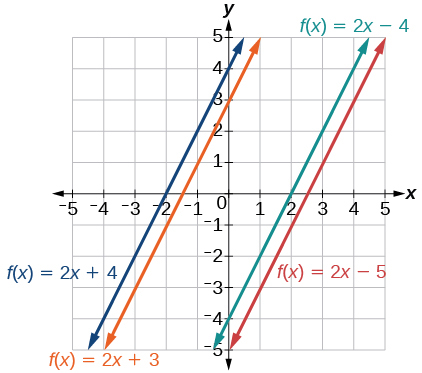## Extensions

Find the value of $\text{\hspace{0.17em}}x\text{\hspace{0.17em}}$ if a linear function goes through the following points and has the following slope: $\text{\hspace{0.17em}}\left(x,2\right),\left(-4,6\right),\text{\hspace{0.17em}}m=3$

Find the value of y if a linear function goes through the following points and has the following slope: $\text{\hspace{0.17em}}\left(10,y\right),\left(25,100\right),\text{\hspace{0.17em}}m=-5$

y = 175

root under 3-root under 2 by 5 y square
The sum of the first n terms of a certain series is 2^n-1, Show that , this series is Geometric and Find the formula of the n^th
cosA\1+sinA=secA-tanA
why two x + seven is equal to nineteen.
The numbers cannot be combined with the x
Othman
2x + 7 =19
humberto
2x +7=19. 2x=19 - 7 2x=12 x=6
Yvonne
because x is 6
SAIDI
what is the best practice that will address the issue on this topic? anyone who can help me. i'm working on my action research.
simplify each radical by removing as many factors as possible (a) √75
how is infinity bidder from undefined?
what is the value of x in 4x-2+3
give the complete question
Shanky
4x=3-2 4x=1 x=1+4 x=5 5x
Olaiya
hi can you give another equation I'd like to solve it
Daniel
what is the value of x in 4x-2+3
Olaiya
if 4x-2+3 = 0 then 4x = 2-3 4x = -1 x = -(1÷4) is the answer.
Jacob
4x-2+3 4x=-3+2 4×=-1 4×/4=-1/4
LUTHO
then x=-1/4
LUTHO
4x-2+3 4x=-3+2 4x=-1 4x÷4=-1÷4 x=-1÷4
LUTHO
A research student is working with a culture of bacteria that doubles in size every twenty minutes. The initial population count was  1350  bacteria. Rounding to five significant digits, write an exponential equation representing this situation. To the nearest whole number, what is the population size after  3  hours?
v=lbh calculate the volume if i.l=5cm, b=2cm ,h=3cm
Need help with math
Peya
can you help me on this topic of Geometry if l help you
litshani
( cosec Q _ cot Q ) whole spuare = 1_cosQ / 1+cosQ
A guy wire for a suspension bridge runs from the ground diagonally to the top of the closest pylon to make a triangle. We can use the Pythagorean Theorem to find the length of guy wire needed. The square of the distance between the wire on the ground and the pylon on the ground is 90,000 feet. The square of the height of the pylon is 160,000 feet. So, the length of the guy wire can be found by evaluating √(90000+160000). What is the length of the guy wire?
the indicated sum of a sequence is known as
how do I attempted a trig number as a starter
cos 18 ____ sin 72 evaluateByByBy Dewey ComptonBy RhodesBy Sarah WarrenBy Madison ChristianBy Cath YuBy Madison ChristianBy OpenStaxBy Anonymous UserBy Brooke DelaneyBy Katie Montrose